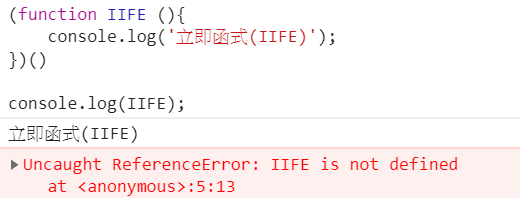0

# 這個章節要來討論立即函式(IIFE)

``````function FnA () {
console.log('something');
}

FnA();
``````

``````(function () {
console.log('第一種，小括號在函式結尾的後面');
}());

(function() {
console.log('第二種，小括號在外面');
})();
``````

## 立即函式(IIFE)的重點

1. 立刻執行
2. 無法在函式外再次被執行
``````(function IIFE (){
console.log('立即函式(IIFE)');
})()

console.log(IIFE);
``````## 透過立即函式來限制變數的作用域

``````(function () {
var Ming = '小明';
console.log(Ming); // 小明
})();

console.log(Ming); // not define
``````

## 傳參數到立即函式中

``````var arg = '我是參數';
(function (parameter) {
var Ming = '小明';
console.log(Ming + parameter); // 小明我是參數
})(arg);
``````

## 立即函式回傳值

``````var whereMing = (function (where) {
console.log('where', where);
return where;
})('小明在西門');

console.log(whereMing); // 小明在西門
``````

## 立即函式之間的共同變數溝通

### 方法1: 全域變數

``````var a = {};
(function (b) {
b.person = '小明';
})(a);

(function (c) {
console.log(c.person); // 小明
})(a);
``````

### 方法1: 全域屬性

``````(function (global) {
global.person = '小明';
})(window);

(function () {
console.log(person); // 小明
})();
``````